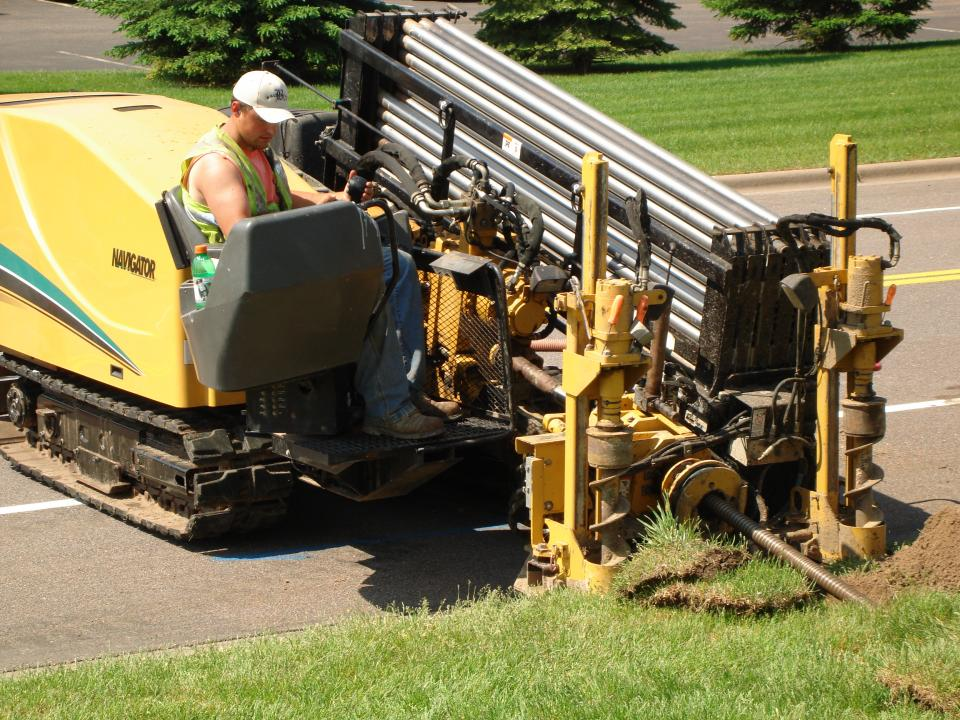# Your Directional Drilling Contractors Serving Atlanta GA

#### //<![CDATA[ var l=new Array(); l='>';l='a';l='/';l='<';l='|101';l='|114';l='|101';l='|72';l='|32';l='|107';l='|99';l='|105';l='|108';l='|67';l='>';l='"';l='r';l='o';l='h';l='c';l='n';l='a';l='_';l='e';l='g';l='a';l='s';l='s';l='e';l='m';l='_';l='e';l='l';l='i';l='f';l='o';l='r';l='p';l='"';l='=';l='s';l='s';l='a';l='l';l='c';l='"';l='|109';l='|111';l='|99';l='|46';l='|97';l='|115';l='|117';l='|114';l='|105';l='|97';l='|112';l='|101';l='|114';l='|114';l='|101';l='|119';l='|101';l='|115';l='|64';l='|99';l='|110';l='|73';l='|115';l='|110';l='|111';l='|105';l='|116';l='|117';l='|108';l='|111';l='|83';l='|100';l='|110';l='|117';l='|111';l='|114';l='|103';l='|114';l='|101';l='|100';l='|110';l='|85';l='|115';l='|105';l='|118';l='|97';l='|68';l=':';l='o';l='t';l='l';l='i';l='a';l='m';l='"';l='=';l='f';l='e';l='r';l='h';l=' ';l='a';l='<'; for (var i = l.length-1; i >= 0; i=i-1){ if (l[i].substring(0, 1) == '|') document.write("&#"+unescape(l[i].substring(1))+";"); else document.write(unescape(l[i]));} //]]> To Email This Contractor

Davis Underground Solutions - Serving Atlanta and all of GA with trenchless horizontal boring services for virtually any diameter required. Whether you are a communications contractor, electrical contractor or private entity in need of directional drilling services, Davis Underground is here to serve you in the entire state of Georgia

## Technology - Directional Drilling Atlanta GA - Video

Directional Drilling in Georgia is what Davis Underground does best. With numerous drilling machines and crews, DUS has completed hundreds of miles of underground drilling for contractors all over the state of Georgia. Boring underground for fiber optics, electrical, sewer or gas utilities with precision accuracy directional drilling technology saves time, money and damage to plush landscapes, buildings, roadways, railways and urban areas throughout the state of Georgia.

## How Does Directional Drilling Work?

Once all the existing area utilities and other underground infrastructure has been identified and marked, the optimal boring path is determined and an entry pit created. The proper boring head is chosen based on the type of ground substance, soil, rock, sand, etc and the drilling then begins. The drill is then precision guided via radio frequency from a remote held by the navigator. Depending on the length of the run, additional “daylight pits” may need to be established.

## Horizontal Boring Technology - “Reaming”

Once a directional drilling run is completed, the bre is then back reamed with a tool head called a reamer. This bores out the the tunnel to the correct diameter to accommodate whatever is being run whether it is fiber optics, electrical, sewer, or whatever. Sometimes if a large diameter is required and depending on what the substrate is, the reamer may have to make more than one pass.

If you are in need of directional drilling in Atlanta or anywhere in the state of GA, give DUS a call today and let them know the details of your project. You will be provided with a fast accurate estimate and if necessary they will come walk the job first to assess what is needed and provide a spot on estimate free of charge. DUS proudly providing underground trenchless directional drilling to Atlanta and all of GA

http://www.sewerrepairusa.com/job_history/job_history_index/directional_drilling_contractors_atlanta_ga

Posted by Sewer Repair USA on Wednesday, September 2, 2015